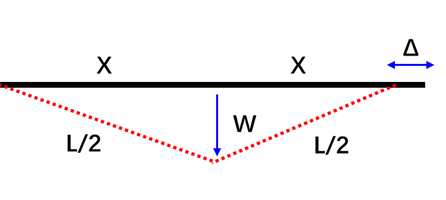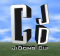# [几何][数学] 梁跨中下挠引起的端部水平位移有多大？(How large is the end horizontal displacement caused by the midspan deflection of the beam?)$$\Delta = L – 2X$$

$$2X = 2\sqrt {{{\left( {\frac{L}{2}} \right)}^2} – {\omega ^2}}$$

$$\begin{array}{l} \Delta = L – 2X\\ = L – 2\sqrt {{{\left( {\frac{L}{2}} \right)}^2} – {\omega ^2}} \\ = L – 2\sqrt {{{\left( {\frac{L}{2}} \right)}^2}\left( {1 – {\omega ^2}{{\left( {\frac{2}{L}} \right)}^2}} \right)} \\ = L – L\sqrt {\left( {1 – {{\left( {\frac{{2\omega }}{L}} \right)}^2}} \right)} \end{array}$$

$$\sqrt {1 – x} \approx 1 – \frac{1}{2}x$$

$$\sqrt {\left( {1 – {{\left( {\frac{{2\omega }}{L}} \right)}^2}} \right)} \approx 1 – \frac{1}{2}{\left( {\frac{{2\omega }}{L}} \right)^2}$$

$$\begin{array}{l} \Delta = L – L\sqrt {\left( {1 – {{\left( {\frac{{2\omega }}{L}} \right)}^2}} \right)} \\ \approx L – L\left( {1 – \frac{1}{2}{{\left( {\frac{{2\omega }}{L}} \right)}^2}} \right)\\ \approx 2\frac{{{\omega ^2}}}{L} \end{array}$$

• 相关博文( Related Topics)

 [工具][软件][规范] 广东省标准《高层建筑混凝土结构设计规范》反应谱计算工具

 [结构力学][结构设计] 两端固支梁弯矩为0点距端部的距离

 [抗震][结构设计][规范] 非抗震设计情况下混凝土柱的“轴压比”可达多大？

 [结构设计][动力学] YJK中CQC振型组合地震力的复核

 [结构设计][楼梯] 混凝土楼梯施工图笔记

 [结构][设计][规范] 关于结构倾覆力矩计算公式的另一种理解

 [结构设计][规范] 结构整体倾覆力矩及抗倾覆力矩的计算——以YJK为例

 [YJK][结构设计] YJK中的地下室侧土侧向约束土弹簧测试

 [结构设计][规范] 关于“扭转耦联”、“偶然偏心”、“双向地震作用”的总结

 [结构设计][规范] 与“嵌固”相关的规范条文总结

 [抗震][结构设计] 规范的各种刚度比”Ratx,Ratx1,Ratx2,RJX1,RJX3″及嵌固层

 [抗震][结构设计] 关于“扭转效应明显”与“两个水平方向振型参与系数”

 [结构设计] [超限设计] 规范“扭转位移比”的相关条文

 [结构设计][地震作用][规范]振型分解反应谱法的一些概念总结 (Basic Concepts of Response Spectra Method)

 [抗震][设计] 关于地下室的抗震等级如何取？

 [钢结构][笔记] 钢梁的局部稳定与腹板加劲肋 (Local Stability of Steel Beam and Web Stiffeners)

 [结构设计] 特一级剪力墙、筒体墙的设计规定

 [结构设计][抗震][笔记] 什么是规定水平力？

 [结构设计][超限][工具] “高规”结构抗震性能目标查询工具

 [结构设计] 开斜洞剪力墙的受力特性

 [结构设计][规范] 什么是重力荷载代表值？

 [抗震设计][结构规范] 如何有效考虑结构在地震作用下的“扭转影响”？！

 [抗震设计][结构规范] 规定水平力、倾覆弯矩、振型组合等电算结果的复核总结

 [地震][结构] 双向地震作用效应,【先振型组合,再方向组合】及【先方向组合再方向组合】的差异？(实际案例测算)

 [规范][结构][设计] 剪力墙边缘构件的尺度问题 (The dimension problem of the boundary element of the shear wall)

 [结构][设计][规范] 关于结构倾覆力矩计算公式的另一种理解

 [结构][YJK][设计] “一次性加载”、“模拟施工1加载”及“模拟施工3加载”的差别及案例测算 ( Construction Simulation)

 [笔记][算例] “剪切型”与”弯曲型”位移曲线的位移角特性

 [抗震][结构设计][规范] 非抗震设计情况下混凝土柱的“轴压比”可达多大？

 [笔记][结构] 斜柱方案受力分析点

 [结构][笔记][材料] 矩形截面与H形截面的抗弯能力 ( Bending Resistance of Rectangular Section and H-shaped Section)

 [笔记][结构][设计] 钢管与钢管混凝土截面的刚度差多少？(What is the difference in stiffness between steel tube and concrete-filled steel tube section)

 [结构力学][结构设计] 两端固支梁弯矩为0点距端部的距离？

 [几何][数学] 梁跨中下挠引起的端部水平位移有多大？(How large is the end horizontal displacement caused by the midspan deflection of the beam?)

 [结构设计][笔记] 钢筋桁架楼承板和压型钢板组合楼板

 [笔记][规范][钢结构] 美国规范中的ASD及LRFD

 [笔记][算例][减振] 调谐液体阻尼器减震算例 [Example of Vibration Reduction of Tuned Liquid Damper (TLD) ]

 [抗震][消能减震] 结构应变能计算公式 (Structural Strain Energy)

 [结构力学][笔记] 不同支座条件的斜梁的受力分析(两道结构力学题)

 [抗震][动力学] 对于整体结构，X向地震作用下有Y向剪力吗？有！！

 [结构][软件] 刚度越大内力越大吗？(单榀框架测算)

 [结构][施工现场] 液压锤击预应力管桩施工见闻

 为何结构越长温度效应越显著？？

 为何长的橡皮筋比短的橡皮筋更容易拉伸？

 [FEM][SteelStructures] 有侧移与无侧移框架的屈曲模态 (Buckling Modes of Frames with and without Sidesway)

 [结构][有限元][PFSAP] 平行弦桁架简单算例及概念 ( Basic concepts and examples of parallel chord truss)

 [钢结构][软件] 格构柱缀条布置方案力学概念测算对比 (Lace Bar Arrangement Patterns in Steel Lattice Column)

• 微信公众号 ( Wechat Subscription)

### 4 thoughts on “[几何][数学] 梁跨中下挠引起的端部水平位移有多大？(How large is the end horizontal displacement caused by the midspan deflection of the beam?)”

1.有意思的问题。简单计算的话，截面形心轴所在轴线处的轴向位移为零，支座截面底部出现向外轴向位移，位移值大约为梁端转角乘以形心高度。

•的确是

2.•